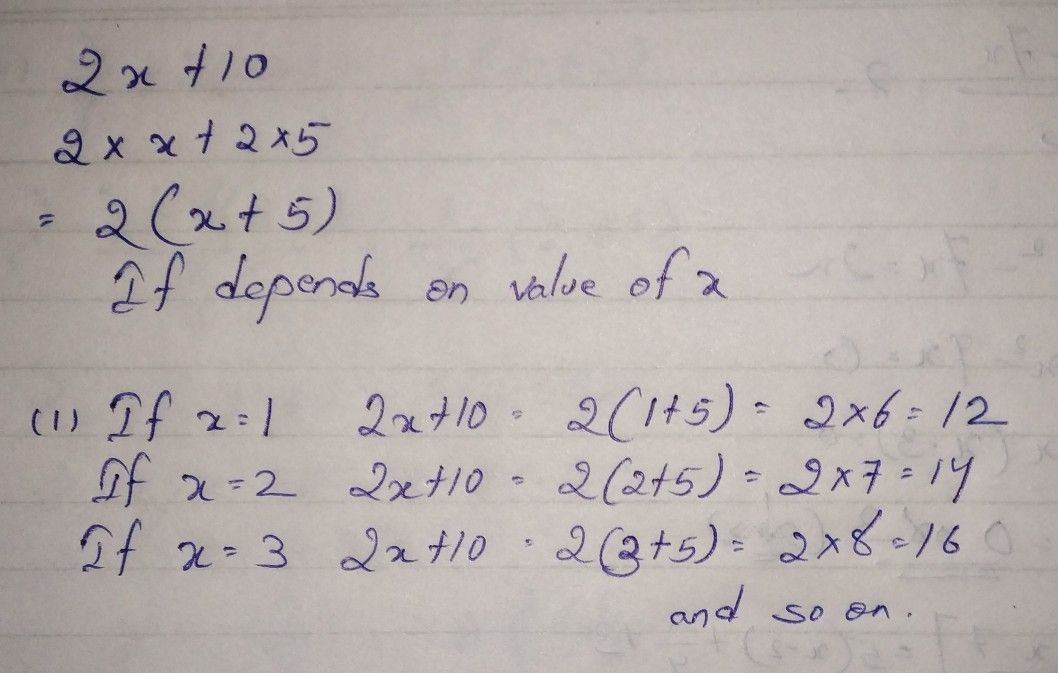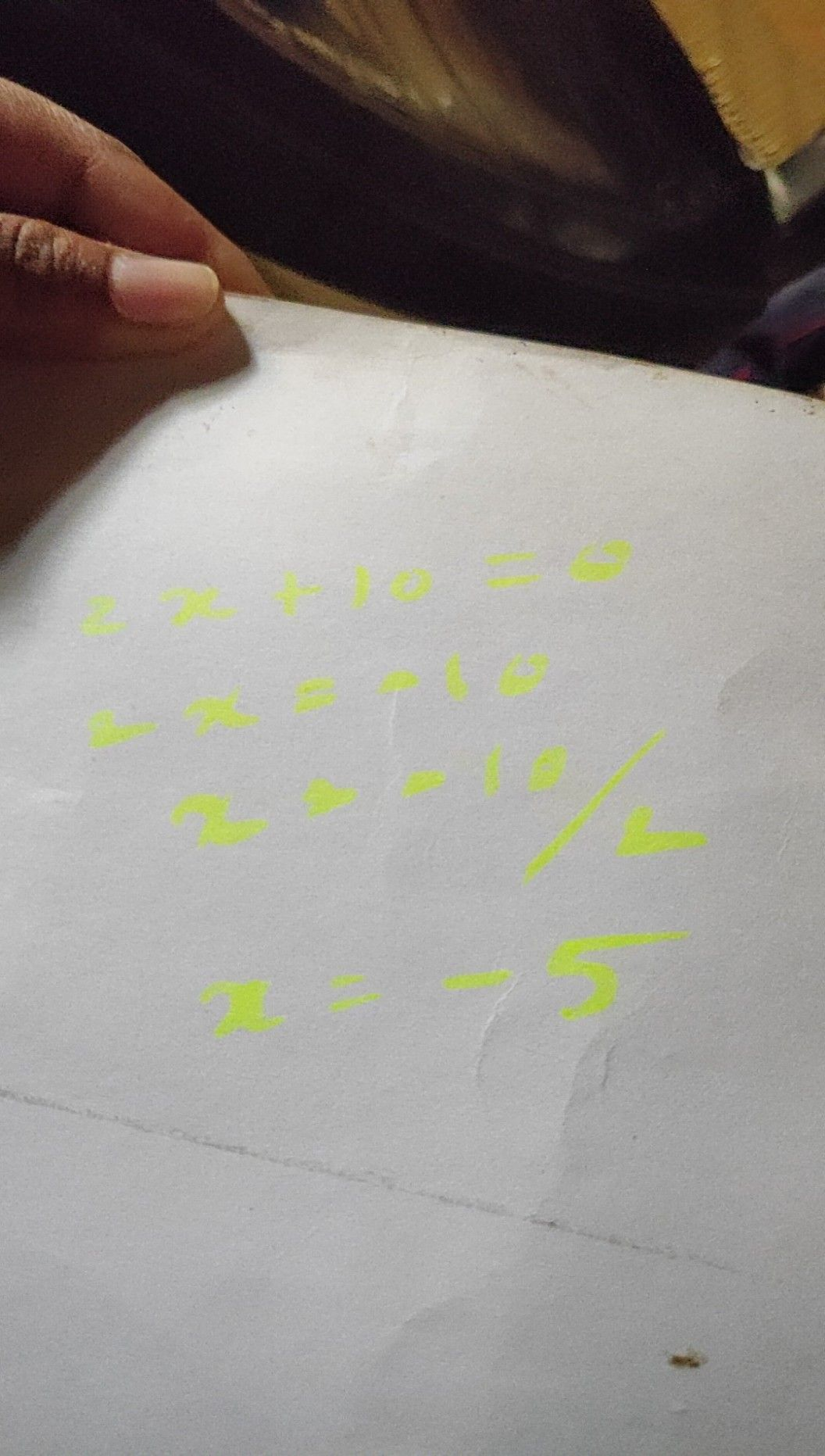Symbol
Problem$25x=0=2$
1st-6th grade
Algebra
SolutionQanda teacher - SaumyajeetStudent
how is it possible
factors are impossibleQanda teacher - Saumyajeet
whyStudentQanda teacher - Saumyajeet
if the equation equates to zero then x=-5
but in question it is asked what is 2x+10
i mean 2x+10=?